Study Materials: ncert solutions

Our ncert solutions for Exercise 3.7 Class 6 maths 3. Playing with Numbers - ncert solutions - Toppers Study is the best material for English Medium students cbse board and other state boards students.

Solutions ⇒ Class 6th ⇒ Mathematics ⇒ 3. Playing with Numbers

# Exercise 3.7 Class 6 maths 3. Playing with Numbers - ncert solutions - Toppers Study

Topper Study classes prepares ncert solutions on practical base problems and comes out with the best result that helps the students and teachers as well as tutors and so many ecademic coaching classes that they need in practical life. Our ncert solutions for Exercise 3.7 Class 6 maths 3. Playing with Numbers - ncert solutions - Toppers Study is the best material for English Medium students cbse board and other state boards students.

## Exercise 3.7 Class 6 maths 3. Playing with Numbers - ncert solutions - Toppers Study

CBSE board students who preparing for class 6 ncert solutions maths and Mathematics solved exercise chapter 3. Playing with Numbers available and this helps in upcoming exams 2023-2024.

### You can Find Mathematics solution Class 6 Chapter 3. Playing with Numbers

• All Chapter review quick revision notes for chapter 3. Playing with Numbers Class 6
• NCERT Solutions And Textual questions Answers Class 6 Mathematics
• Extra NCERT Book questions Answers Class 6 Mathematics
• Importatnt key points with additional Assignment and questions bank solved.

NCERT Solutions do not only help you to cover your syllabus but also will give to textual support in exams 2023-2024 to complete exercise 3.7 maths class 6 chapter 3 in english medium. So revise and practice these all cbse study materials like class 6 maths chapter 3.7 in english ncert book. Also ensure to repractice all syllabus within time or before board exams for ncert class 6 maths ex 3.7 in english.

See all solutions for class 6 maths chapter 3 exercise 3 in english medium solved questions with answers.

### Exercise 3.7 class 6 Mathematics Chapter 3. Playing with Numbers

• Exercise 3.7 Class 6 Maths 3. Playing With Numbers - Ncert Solutions - Toppers Study
• Class 6 Ncert Solutions
• Solution Chapter 3. Playing With Numbers Class 6
• Solutions Class 6
• Chapter 3. Playing With Numbers Exercise 3.7 Class 6

## Exercise 3.7 Class 6 maths 3. Playing with Numbers - ncert solutions - Toppers Study

#### Exercise: 3.7

Q1. Renu purchases two bags of fertiliser of weights 75 kg and 69 kg. Find the maximum value of weight which can measure the weight of the fertiliser exact number of times.

Solution:

Renu purchases two bags of fertiliser of weights : 75 kg and 69 kg

Therefore, finding prime factorisation of 75 kg and 69 by dividing method: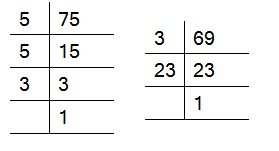75 = 3 × 5 × 5

69 = 3 × 23

Therefore, HCF of (75, 69) = 3

Hence, Maximum Value of weight = 3 kg Answer

Q2. Three boys step off together from the same spot. Their steps measure 63 cm, 70 cm and 77 cm respectively. What is the minimum distance each should cover so that all can cover the distance in complete steps?

Solution: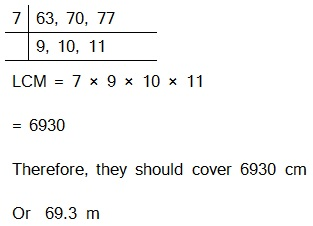Q3. The length, breadth and height of a room are 825 cm, 675 cm and 450 cm respectively. Find the longest tape which can measure the three dimensions of the room exactly.

Solution:

The measurement of longest tape = H.C.F. (825 cm, 675 cm, 450 cm)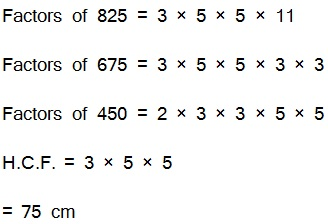Therefore, the longest tape is 75 cm.

Q4. Determine the smallest 3-digit number which is exactly divisible by 6, 8 and 12.

Solution: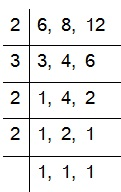L.C.M. of 6, 8 and 12

= 2 × 2 × 2 × 3

= 24

The smallest 3-digit number = 100

To find the number, we have to divide 100 by 24 1

00 = 24 × 4 + 4

Therefore, the required number = 100 + (24 – 4)

= 120.

Q 5. Determine the greatest 3-digit number exactly divisible by 8, 10 and 12.

Solution:

The greatest 3-digit number = 999

Now we find LCM of 8, 10 and 12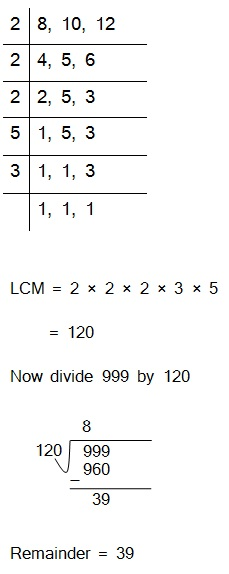So, When we subtract 39 from 999 we get 960.

Which is exactly divisible by 8, 10 and 12.

Hence, The greatest 3-digit number is 960.

Q6. The traffic lights at three different road crossings change after every 48 seconds, 72 seconds and 108 seconds respectively. If they change simultaneously at 7 a.m., at what time will they change simultaneously again?

Solution:

They meet together after LCM (48, 72, 108) seconds.

Now finding LCM,Therefore, LCM (48, 72, 108) = 432

So these three lights burn together after 432 seconds.

432 seconds = 7 minutes 12 second

The time will be 7 a.m. +  7 minutes 12 second

= 7 : 07 : 12 a.m.

7. Three tankers contain 403 litres, 434 litres and 465 litres of diesel respectively. Find the maximum capacity of a container that can measure the diesel of the three containers exact number of times.

Solution:

The maximum capacity of that container = HCF (403, 434, 465)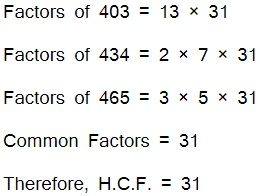Therefore, 31 litres of container is exact number which is required to measure the all containers' diesel.

8. Find the least number which when divided by 6, 15 and 18 leave remainder 5 in each case.

Solution:

The least number will be LCM(6, 15,18) + 5

Therefore, LCM(6, 15, 18)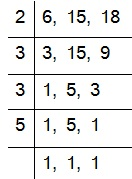LCM = 2 × 3 × 3 × 5

= 90

Now, 90 + 5 = 95

Hence required number 95 which leaves remainder as 5 on dividing by 6, 15, 18.

9. Find the smallest 4-digit number which is divisible by 18, 24 and 32.

Solution: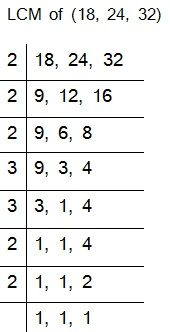LCM = 2 × 2 × 2 × 2 × 2 × 3 × 3

= 288

The smallest 4-digit number = 1000

Now, dividing 1000 by 288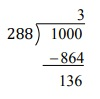Remainder is 136.

1000 needs more (288-136) = 152 to divide exactly by 288

Therefore, Smallest required number is 1000 + 152 = 1152

10. Find the LCM of the following numbers :

(a) 9 and 4

(b) 12 and 5

(c) 6 and 5

(d) 15 and 4

Solution:

(a) 9 and 4

9 and 4 has no common factors

Therefore, LCM = 9 × 4

= 36

Solution:

(b) 12 and 5

12 and 5 has no common factors

Therefore, LCM of 12 and 5 = 12 × 5

= 60

Solution:

(c) 6 and 5

6 and 5 has no common factors

Therefore, LCM of 6 and 5 = 6 × 5

= 30

Solution:

(d) 15 and 4

15 and 4 has no common factors

Therefore, LCM of 15 and 4 = 15 × 4

= 60

Observe a common property in the obtained LCMs. Is LCM the product of two numbers in each case?

Yes, the L.C.M. is equal to the product of two numbers in each case. And L.C.M. is also the multiple of 3 in each case.

11. Find the LCM of the following numbers in which one number is the factor of the other.

(a) 5, 20

(b) 6, 18

(c) 12, 48

(d) 9, 45

Solution:

(a) 5, 20

5 and 20 has common factor 5. Both are divisible by 5 and five is one of them.

Then, then greatest number will be LCM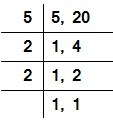LCM = 5 × 2 × 2

Therefore, LCM = 20

Solution:

(b) 6, 18

6 and 18 has common factor 6. and 6 is one of them.

Then the greatest number will be LCM.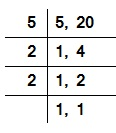LCM = 2 × 3 × 3

Therefore, LCM = 18

Solution:

(c) 12, 48

12 and 48 has common factor 12 and 12 is one of them.

Then the greatest number will be LCM.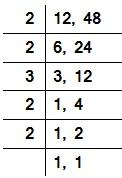LCM = 2 × 2 × 2 × 3

Therefore, LCM = 24

Solution:

(d) 9, 45

9 and 45 has common factor 9 and 45 is one of them.

Then the greatest number will be LCM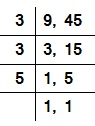LCM = 3 × 3 × 5

Therefore, LCM = 45

What do you observe in the results obtained?

We observe that both numbers belongs to same table and greatest number is the LCM.

Important Study materials for classes 06, 07, 08,09,10, 11 and 12. Like CBSE Notes, Notes for Science, Notes for maths, Notes for Social Science, Notes for Accountancy, Notes for Economics, Notes for political Science, Noes for History, Notes For Bussiness Study, Physical Educations, Sample Papers, Test Papers, Mock Test Papers, Support Materials and Books.Mathematics Class - 11th

NCERT Maths book for CBSE Students.

books

## Study Materials List:

##### Solutions ⇒ Class 6th ⇒ Mathematics
1. Knowing Our Numbers
2. Whole Numbers
3. Playing with Numbers
4. Basic Geometrical Ideas
5. Understanding Elementary Shapes
6. Integers
7. Fractions
8. Decimals

New Books